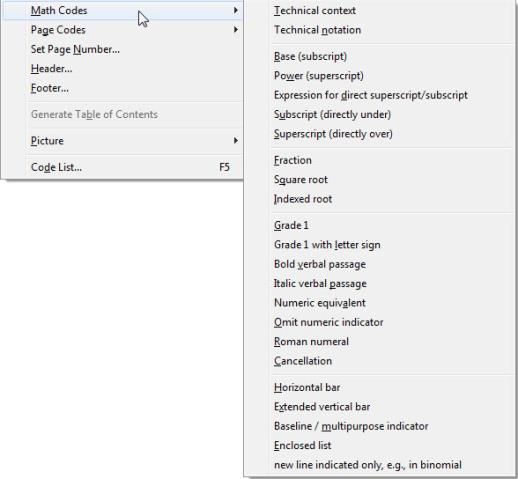### Layout: Math CodesThis menu contains the DBT codes related to translating and formatting mathematics.

Examples of these codes are given in the How-To Guide, a DBT examples document which is typically found in C:\Program Files (x86)\Duxbury\DBT 12.7\Documentation (English)\dochow.dxp.

Unless you are an experienced math braillist, instead of entering math codes directly into DBT you may prefer using a mathematics editor program such as MathType to prepare your material, and then import the resultant file.

MathType is an add-on program for Microsoft Word which facilitates placing mathematical formulae into Word documents. These documents containing MathType equations can be opened in DBT, which will translate them into mathematical braille.

Scientific Notebook, another mathematics editor (no longer being produced), has been a popular program for printed mathematical books, papers, exercises, exam papers, etc. Files produced by version 5.5 of this program can be imported into DBT and converted into Mathematical Braille. Note that only English (American, Nemeth and British) and French Mathematical Braille are supported.

For more details consult the Help topics Scientific Notebook and MathType.

If you want to work in DBT directly, without the assistance of a math editor, there is a basic guide to inserting math codes directly into DBT. See DBT Math Direct Entry.

If you want to work in DBT directly, without the assistance of a math editor, there is a basic guide to inserting math codes directly into DBT. See in the on-line Help: The Menus: Layout: Math Codes: DBT Math Direct Entry.